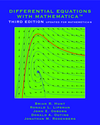Home
Project Description
Authors

Differential Equations
with Mathematica

Differential Equations
with Maple

Differential Equations
with Matlab

## Differential Equations with Mathematica, Third Edition

Table of Contents
 1. Introduction 1 2. Getting Started with Mathematica 7 3. Doing Mathematics with Mathematica 13 4. Using Mathematica Notebooks 31 Problem Set A: Practice with Mathematica 41 5. Solutions of Differential Equations 45 6. A Qualitative Approach to Differential Equations 59 Problem Set B: First Order Equations 69 7. Numerical Methods 79 8. Features of Mathematica 97 Problem Set C:Numerical Solutions of Differential Equations 121 9. Solving and Analyzing Second Order Linear Equations 129 Problem Set D: Second Order Equations 145 10. Series Solutions 159 10. Laplace Transforms 167 Problem Set E: Series Solutions and Laplace Transforms 177 11. Higher Order Equations and Systems of First Order Equations 189 12. Qualitative Theory for Systems of Differential Equations 205 Problem Set F: Systems of Equations 213 Glossary 229 Sample Solutions 239 Index 261